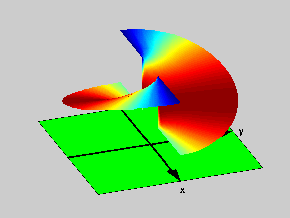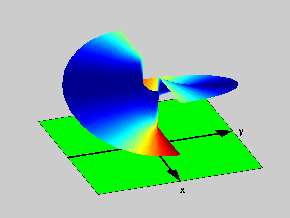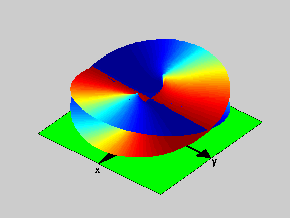18.04 | Fall 1999 | Undergraduate

# Complex Variables with Applications

Study Materials

## Riemann Surfaces: f(z)=(z2-1)1/2

(All images created with MATLAB® software)

Click on pictures for more information and an image of higher resolution.Figure 1: Side view of the Riemann Surface for the function f(z)=(z2-1)1/2

To construct the Riemann Surface for the function f(z)=(z2-1)1/2 we start with the complex plane with two cuts along the real axis: one for x > 1 and the other for x < -1. On this cut plane the function has two branches. Figures 1 & 2 illustrate these branches, which must then be joined (as usual) along the cuts to produce the Riemann Surface. The colors in the pictures are set up to be consistent with the way the branches are to be joined (red to red and blue to blue) along the lips of the branch cuts. Again, as in the case of the  the square root, we must go into the “fourth dimension” to do so.Figure 2: Another Side view of the Riemann Surface for the function f(z)=(z2-1)1/2

That is: the Riemann Surface for f(z)=(z2-1)1/2 is an object in four dimensional space. This surface is shown on Figure 3, where the color coding is the same as that used for Figures 1 & 2 (“Lego” blocks). Notice that Figure 3 is rotated by 90 degrees on the complex plane, relative to the first two figures.Figure 3: Top view of the Riemann Surface for the function f(z)=(z2-1)1/2